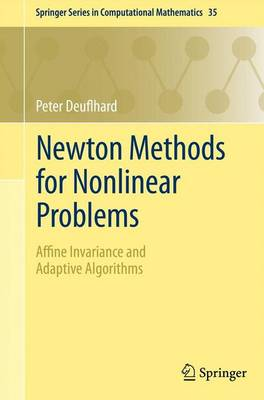Some orders may be delayed by the current Coronavirus lockdown.•# Newton Methods for Nonlinear Problems: Affine Invariance and Adaptive Algorithms - Springer Series in Computational Mathematics 35 (Paperback)

(author)
£54.99
Paperback 424 Pages / Published: 16/09/2011
• We can order this

Usually dispatched within 3 weeks

This book deals with the efficient numerical solution of challenging nonlinear problems in science and engineering, both in finite dimension (algebraic systems) and in infinite dimension (ordinary and partial differential equations). Its focus is on local and global Newton methods for direct problems or Gauss-Newton methods for inverse problems. The term 'affine invariance' means that the presented algorithms and their convergence analysis are invariant under one out of four subclasses of affine transformations of the problem to be solved. Compared to traditional textbooks, the distinguishing affine invariance approach leads to shorter theorems and proofs and permits the construction of fully adaptive algorithms. Lots of numerical illustrations, comparison tables, and exercises make the text useful in computational mathematics classes. At the same time, the book opens many directions for possible future research.

Publisher: Springer-Verlag Berlin and Heidelberg GmbH & Co. KG
ISBN: 9783642238987
Number of pages: 424
Weight: 670 g
Dimensions: 235 x 155 x 22 mm
Edition: 2004

MEDIA REVIEWS

From the reviews:

"This monograph covers a multitude of Newton methods and presents the algorithms and their convergence analysis from the perspective of affine invariance, which has been the subject of research by the author since 1970. ... The book is intended for graduate students of mathematics and computational science and also for researchers in the area of numerical analysis and scientific computing. ... As a research monograph, the book not only assembles the current state of the art, but also points to future research prospects." (Gudula Runger, ACM Computing Reviews, June, 2012)